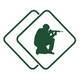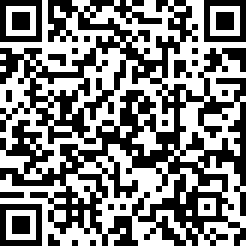#### ASVAB Armed Services Vocational Aptitude Battery (Exam 31)

created by Fisher BRink (@fisher) at Feb. 8, 2016
• What's the sum of the integers from 1 to 300?

• (y2)3 =

• If y = 6, then 2y × y =

• If 0.05x = 1, then x equals:

• 25x2 =

• Factor 9x3 + 18x2 x 2

• Solve for x: 5x + 7 = 6(x 2) 4(2x 3)

• x × x2 =

• (5 + x)2 =

• (3 × 3)(5 3)(6 + 2) = x2. What's the value of x?

• If 5x = 25, x equals:

• Evaluate the expression 6a 3x 2y, if a = 3,x = 7, and y = 4.

• (x + 4)(3x + 5) =

• The cube of 6 is

• In the equation 3x + 7y =21, at what point is the x-axis intersected?

• The average of 54, 61, 70, and 75 is:

• 2 feet 4 inches + 4 feet 8 inches =

• If x = 4, then x4 ÷ x =

• Solve for x: 5 3x 14 + 6x

• (900 × 3) ÷ 6 =

Be the first to review

• info
Quiz Info
• date_range
Feb. 8, 2016, 3:26 a.m.
help_outline
20 questions
dvr
0 completed
remove_red_eye
11 views
people
0 takers
folder•#### Ratings

star_borderstar_borderstar_borderstar_borderstar_border
ratings